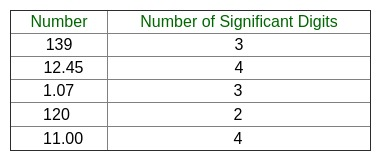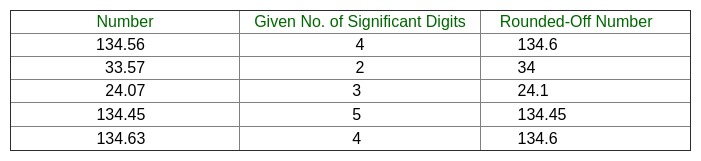Open In App

# Round-off a number to a given number of significant digits

Given a positive number n (n > 1), round-off this number to a given no. of significant digits, d.
Examples:

Input : n = 139.59
d = 4
Output : The number after rounding-off is 139.6 .

The number 139.59 has 5 significant figures and for rounding-off
the number to 4 significant figures, 139.59 is converted to 139.6 .

Input : n = 1240
d = 2
Output : The number after rounding-off is 1200 .

What are significant figures?

Each of the digits of a number that are used to express it to the required degree of accuracy, starting from the first non-zero digit, are called as significant figures.
Since there are numbers with large number of digits, for example,= 3.142857143, so in order to limit such numbers to a manageable number of digits, we drop unwanted digits and this process is called rounding off.
Significant digits include all the digits in a number falling in one of the following categories –

• All non-zero digits.
• Zero digits which-
1. lie between significant digits.
2. lie to the right of decimal point and at the same time to the right of a non-zero digit.
3. are specifically indicated to be significant.

The following table shows numbers and no. of significant digits present in them –Rules for Rounding-off a Number

To round off a number to n significant figures-

1. Discard all digits to the right of nth significant digit.
2. If this discarded number is-
• less than half a unit in nthplace, leave the nth digit unchanged.
• greater than half a unit in the nth place, increase the nth digit by unity.
• exactly half a unit in the nth place, increase the nth digit by unity if its odd, otherwise leave it unchanged.

The following table shows rounding-off a number to a given no. of significant digits –## C++

 // C++ program to round-off a number to given no. of// significant digits#include using namespace std;  // Function to round - off the numbervoid Round_off(double N, double n){    int h;    double l, a, b, c, d, e, i, j, m, f, g;    b = N;    c = floor(N);      // Counting the no. of digits to the left of decimal point    // in the given no.    for (i = 0; b >= 1; ++i)        b = b / 10;      d = n - i;    b = N;    b = b * pow(10, d);    e = b + 0.5;    if ((float)e == (float)ceil(b)) {        f = (ceil(b));        h = f - 2;        if (h % 2 != 0) {            e = e - 1;        }    }    j = floor(e);    m = pow(10, d);    j = j / m;    cout << "The number after rounding-off is " << j;}  // Driver main functionint main(){    double N, n;      // Number to be rounded - off    N = 139.59;      // No. of Significant digits required in the no.    n = 4;      Round_off(N, n);    return 0;}

## Java

 // Java program to round-off a number to given no. of// significant digits  import java.io.*;import static java.lang.Math.*;public class A {      // Function to round - off the number    static void Round_off(double N, double n)    {        int h;        double l, a, b, c, d, e, i, j, m, f, g;        b = N;        c = floor(N);          // Counting the no. of digits to the left of decimal point        // in the given no.        for (i = 0; b >= 1; ++i)            b = b / 10;          d = n - i;        b = N;        b = b * pow(10, d);        e = b + 0.5;        if ((float)e == (float)ceil(b)) {            f = (ceil(b));            h = (int)(f - 2);            if (h % 2 != 0) {                e = e - 1;            }        }        j = floor(e);        m = pow(10, d);        j = j / m;        System.out.println("The number after rounding-off is "                           + j);    }      // Driver main function    public static void main(String args[])    {        double N, n;          // Number to be rounded - off        N = 139.59;          // No. of Significant digits required in the no.        n = 4;          Round_off(N, n);    }}

## Python3

 # Python 3 program to round-off a number # to given no. of significant digitsfrom math import ceil, floor, pow  # Function to round - off the numberdef Round_off(N, n):    b = N    c = floor(N)      # Counting the no. of digits     # to the left of decimal point     # in the given no.    i = 0;    while(b >= 1):        b = b / 10        i = i + 1      d = n - i    b = N    b = b * pow(10, d)    e = b + 0.5    if (float(e) == float(ceil(b))):        f = (ceil(b))        h = f - 2        if (h % 2 != 0):            e = e - 1    j = floor(e)    m = pow(10, d)    j = j / m    print("The number after rounding-off is", j)  # Driver Codeif __name__ == '__main__':          # Number to be rounded - off    N = 139.59      # No. of Significant digits     # required in the no.    n = 4      Round_off(N, n)  # This code is contributed by# Surendra_Gangwar

## C#

 // C# program to round-off a number // to given no. of significant digitsusing System;  class A {      // Function to round - off the number    static void Round_off(double N, double n)    {        int h;        double b, d, e, i, j, m, f;        b = N;        // c = Math.Floor(N);          // Counting the no. of digits to the        // left of decimal point in the given no.        for (i = 0; b >= 1; ++i)            b = b / 10;          d = n - i;        b = N;        b = b * Math.Pow(10, d);        e = b + 0.5;        if ((float)e == (float)Math.Ceiling(b)) {            f = (Math.Ceiling(b));            h = (int)(f - 2);            if (h % 2 != 0) {                e = e - 1;            }        }        j = Math.Floor(e);        m = Math.Pow(10, d);        j = j / m;        Console.WriteLine("The number after " +                        "rounding-off is " + j);    }      // Driver main function    public static void Main()    {        double N, n;          // Number to be rounded - off        N = 139.59;          // No. of Significant digits required in the no.        n = 4;          Round_off(N, n);    }}  // This code is contributed by vt_m.

## PHP

 = 1; ++$i) $b = $b / 10;   $d = $n - $i;    $b = $N;    $b = $b * pow(10, $d); $e = $b + 0.5; if ($e == ceil($b))  { $f = (ceil($b)); $h = $f - 2; if ($h % 2 != 0)         {            $e = $e - 1;        }    }    $j = floor($e);    $m = pow(10, $d);    $j = $j / $m; echo "The number after rounding-off is " ,$j;}      // Driver Code    $N; $n;      // Number to be rounded - off    $N = 139.59;   // No. of Significant digits // required in the no. $n = 4;      Round_off($N, $n);  // This code is contributed by anuj_67?>

## Javascript

 

Output:

The number after rounding-off is 139.6

Time complexity: O(logN)

Space complexity: O(1)
This article is contributed by Mrigendra Singh. If you like GeeksforGeeks and would like to contribute, you can also write an article using write.geeksforgeeks.org or mail your article to review-team@geeksforgeeks.org. See your article appearing on the GeeksforGeeks main page and help other Geeks.